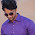# Java code to find sum of given series using recursion : 1^2 + 2^2 + 3^2 +.......+ n^2

Java program to find the sum of the following series using recursion : 12 + 22 + 32 +.......+ n2

```import java.io.*;
class Series
{
public static void main(String args[]) throws IOException
{
Series call = new Series();
System.out.print("Enter value of n : ");
int n = Integer.parseInt(br.readLine());
System.out.println("Sum of series = " +call.sum(n));
}
int sum(int n)
{
if(n==1)
return 1;
else
return (n*n)+sum(n-1);
}
}```

ALGORITHM:-

1. Start

2. Accept a number n from user.

3. Using method of recursion, add all the squared numbers upto n.

4. Print the sum.

5. End

OUTPUT:-

Enter value of n : 5

Sum of series = 55

Related Programs:-

Find the pig Latin equivalent of a word

Change Lowercase letters to Uppercase and vice-versa in a string

Check whether a string is a Palindrome or not

Check the number of Uppercase letters, Lowercase letters, numerals, vowels, spaces & special characters in a string

Read the date, day and year

1.2.